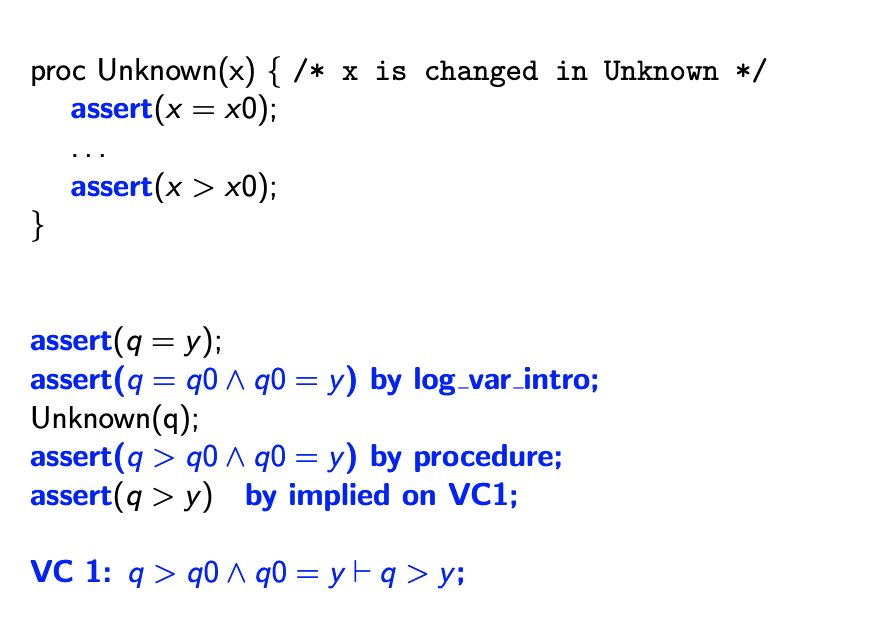SE212

# Program Correctness §

The goal of program correctness is to discover subtle bugs in a program and then prove that a program has the correct behaviour once all the bugs have been fixed.

But what is “correct behaviour”?

• Correctness is a relative concept (i.e., relative to the specification).

• Using proof rules for the constructs of a programming language, we prove that a program satisfies its specification.

• Partial Correctness: Partial correctness means that the program fulfills its specification, or does not terminate (ex: infinite loop or recursion).

• Proof of partial correctness checks that the postcondition is true if the program terminates
• Total Correctness = Partial Correctness + Termination

• Proof of total correctness shows that the loop terminates

The program below is partially correct, because it never terminates: (see Program Specification for syntax)

assert(true); # precondition
while true do { # program
X := 0;
}
assert(X > 0); #postcondition

We use Floyd-Hoare Logic for the Proof Theory to show Program Correctness.

### Templates of Proofs §

In program correctness proofs, we often work backwards from the postcondition.

• This is important, we need to start from the assertion that they give us. You cannot conjure up any assertions. Even saying assert(x +1 = x+ 1), you need to build on something from before, use by implied using arith or by implied using VC1

For example,

assert(????);
X := Y;
assert(X > 0);

# This would be
assert(Y > 0);
X := Y;
assert(X > 0);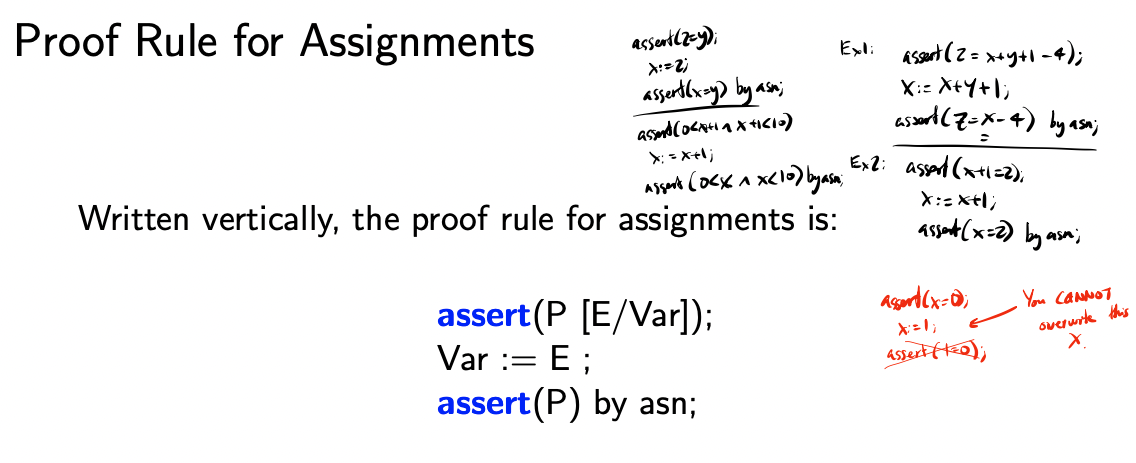Reminder: We define to be the formula obtained by replacing in every free occurrence of variable with .

• The original expression CAN have the variable , but there shouldn’t be a Variable Capture (see note for example)

You have to be really careful, it’s an assignment operator, so you cannot just do this (see Variable Capture):

assert(x=0);
x := 1;
assert(1 = 0); # Because x is not free 

One thing I got super confused about, and then I was doing the assignment, was like, do I need to replace everything? And the answer is no. For example:

assert(true);
assert(x + x+ x = 3 * x) by implied using arith; # P[x/y], there CANNOT be any y's here
y := x; # y = x
assert((x+x) + y = 3 * x) by asn; # P, notice that all the x are still there
• Basically, just think about it intuitively. You don’t want to overwrite variables, which is why the conditions must be free. If you flip the assertions around, you would be overwriting the variable, so the assertion would no longer hold
• Also think of replacing y := x with x := y, that would not work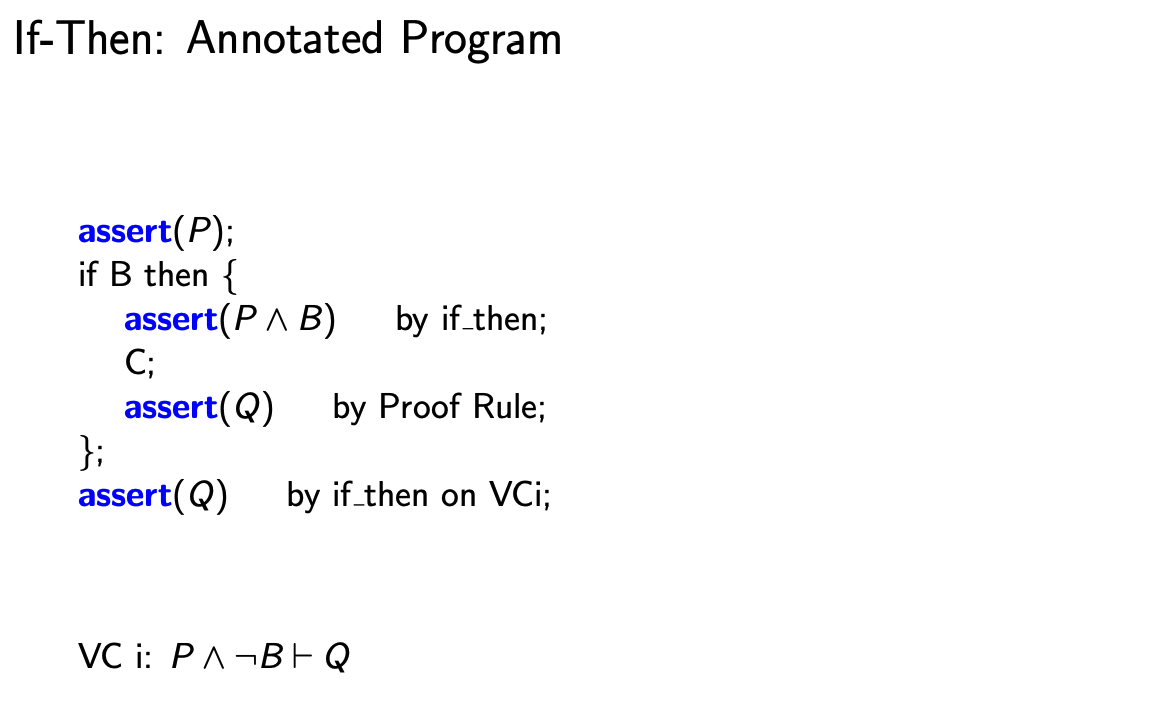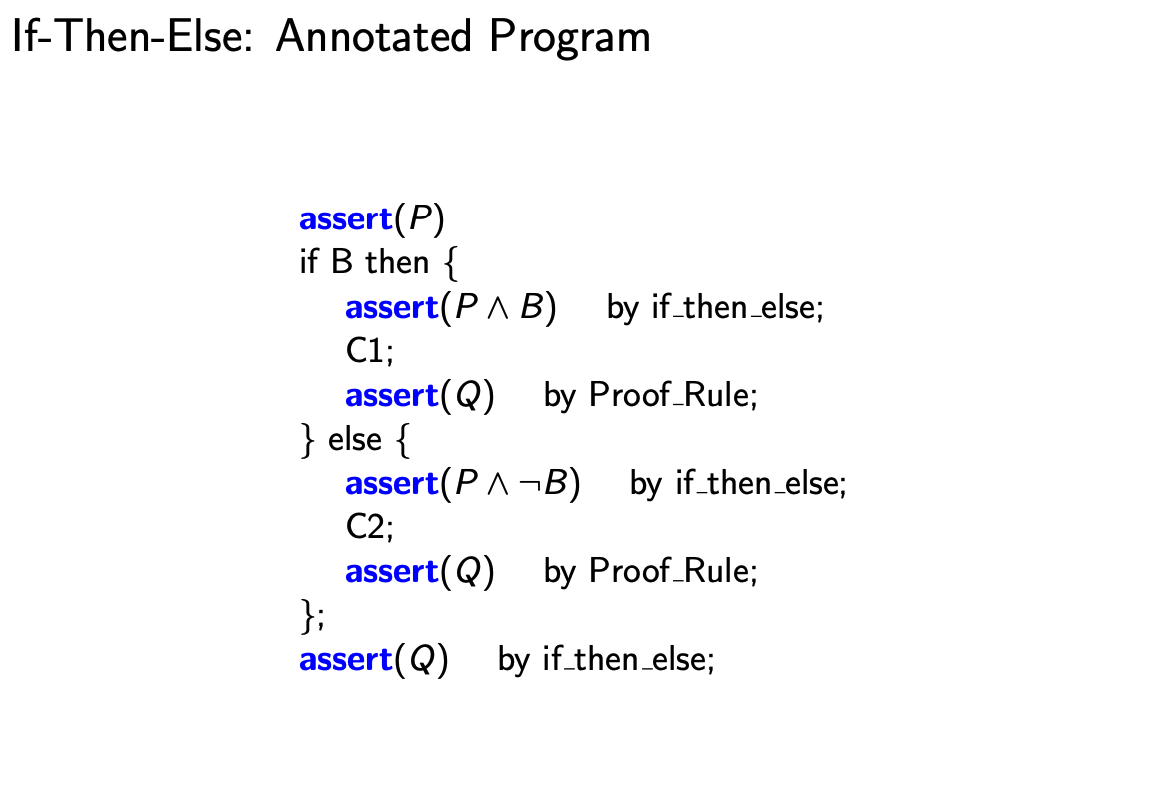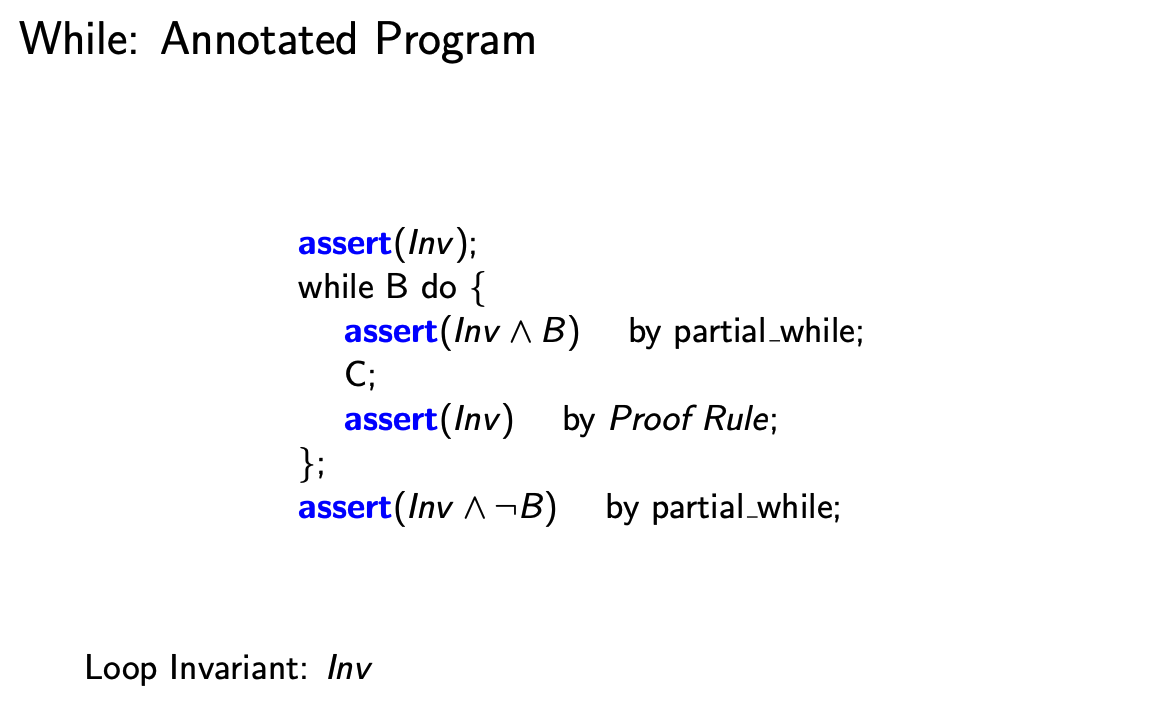Review on Binary Relation.

We can make use of arrays in 2 ways:

1. A reference to a value in an array (e.g., on RHS of an assignment or in a condition)
• You can use by asn
2. Assignments to an element of an array (on LHS of an assignment)
• NEW, use by array_asn, see below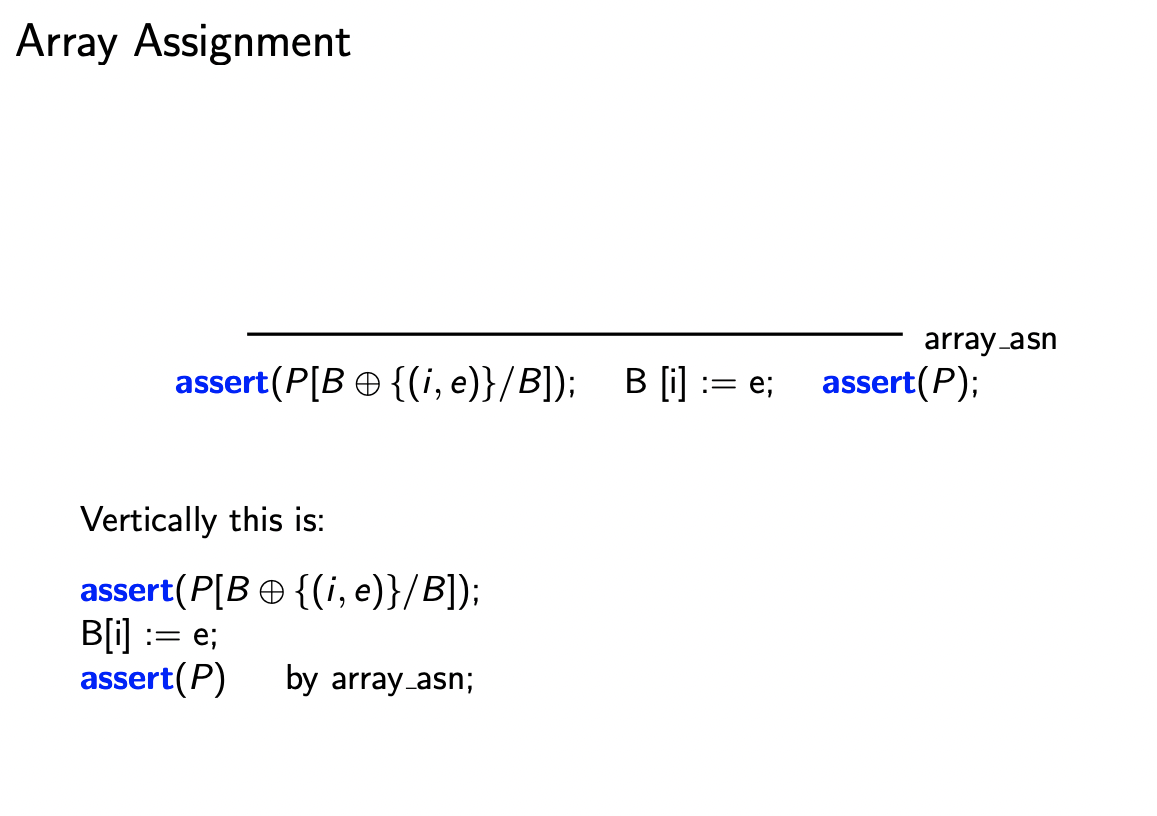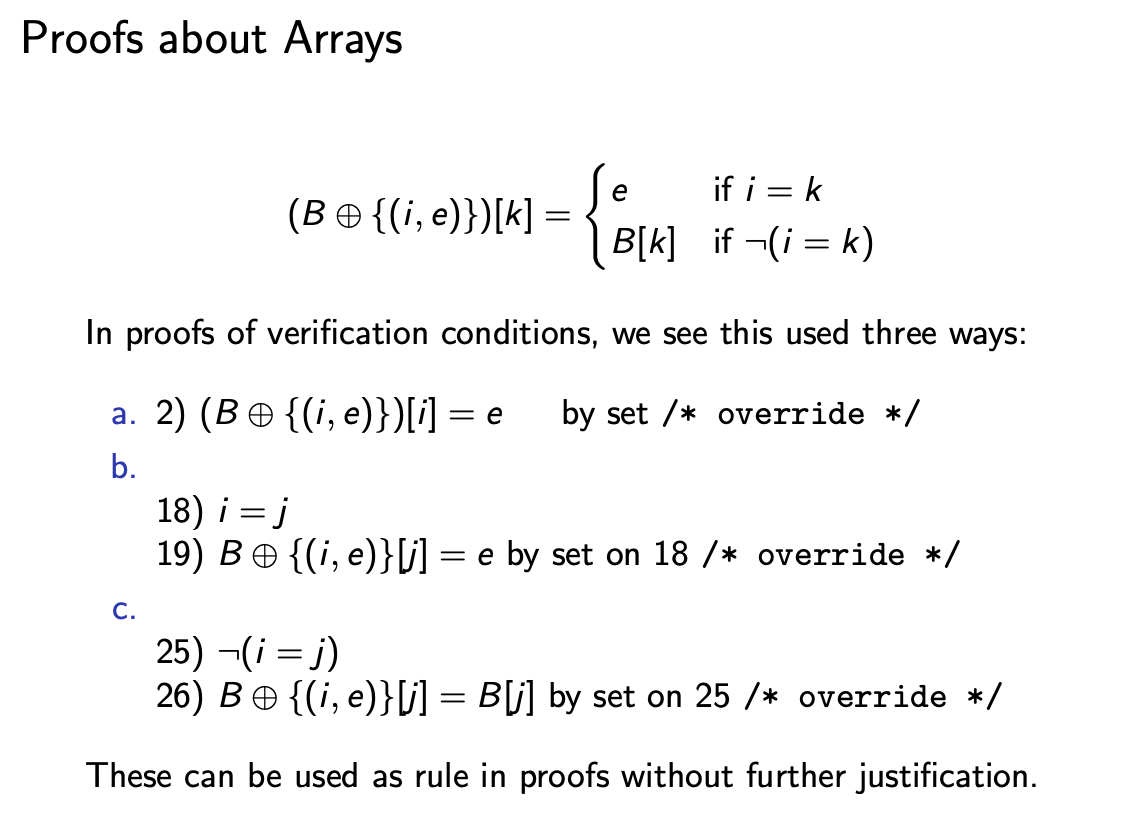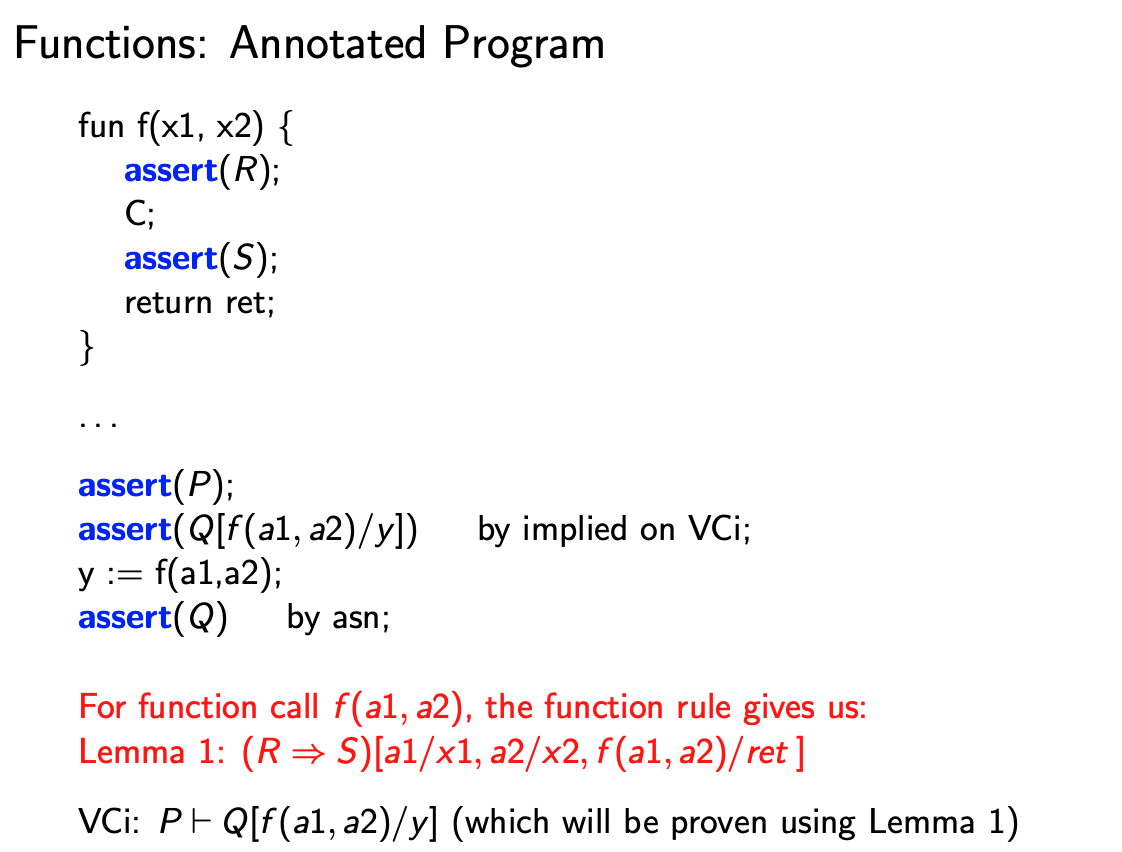You need to make use of the function rule.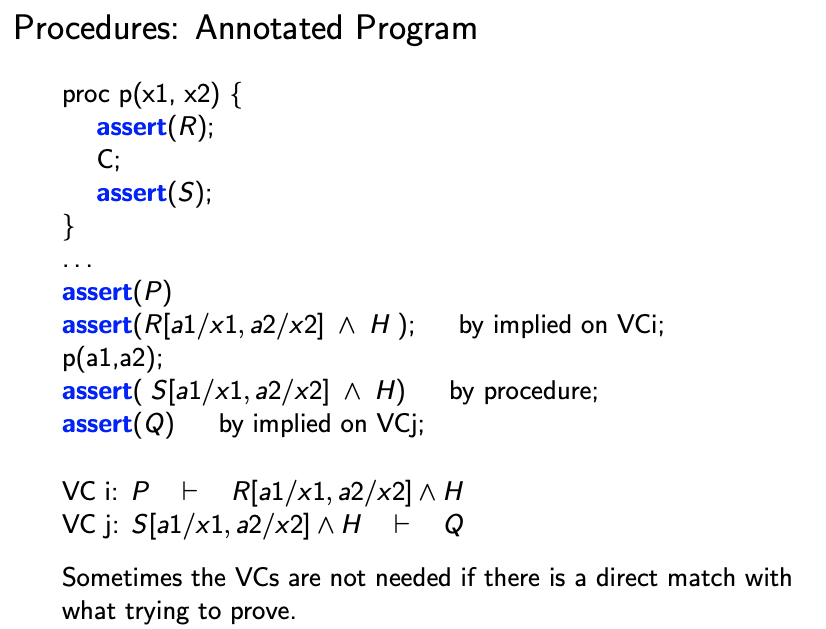Log_var_intro, this is something fairly new that I haven’t seen too much, but it is worthwhile